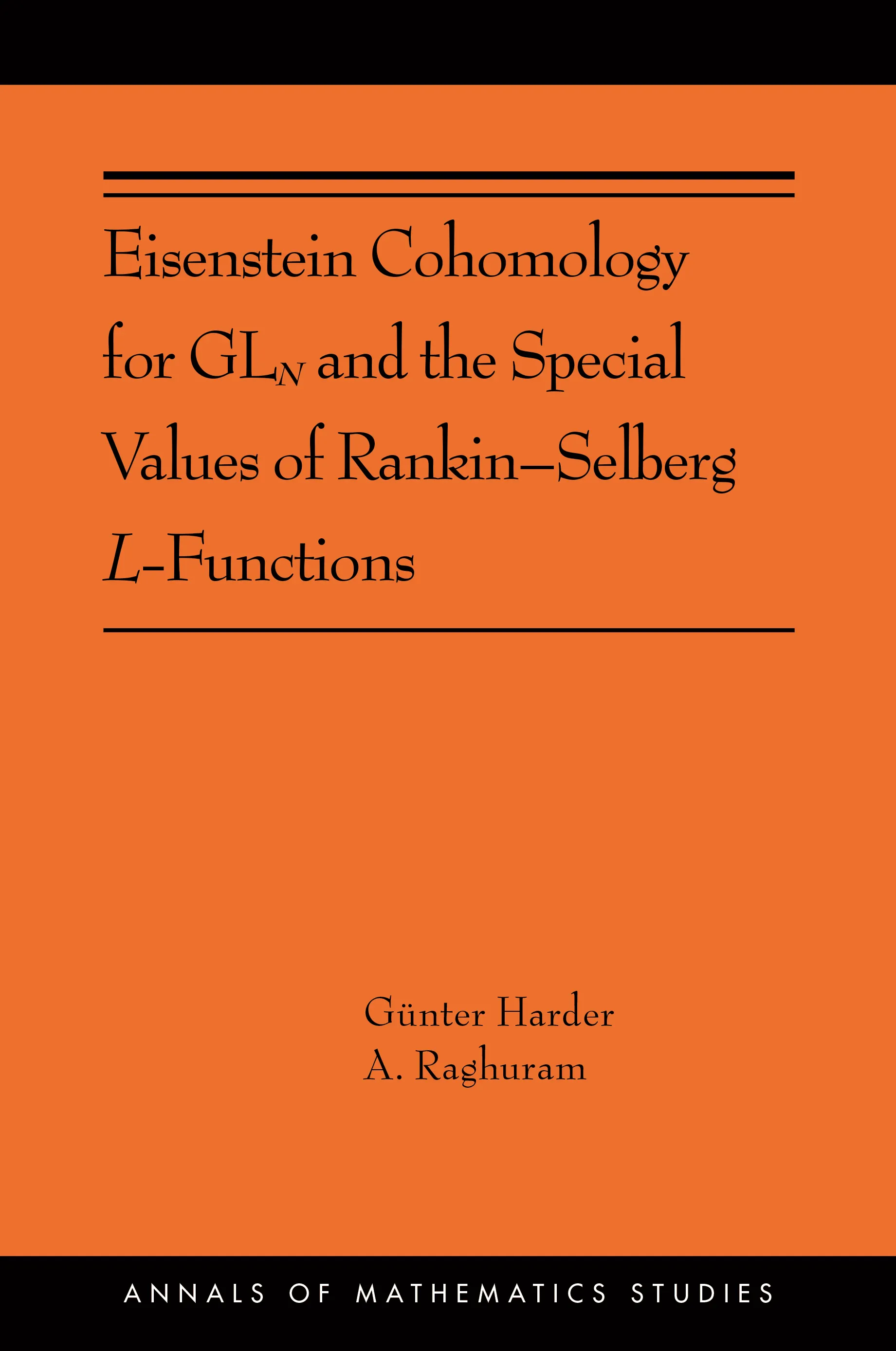#### Eisenstein Cohomology for GLN and the Special Values of Rankin–Selberg L-Functions

Günter Harder

50% off with code MAY50

# Eisenstein Cohomology for GLN and the Special Values of Rankin–Selberg L-Functions: (AMS-203)## Paperback

50% off with code MAY50

Sale Price:
\$37.50/£31.00
Price:
\$75.00/£62.00
ISBN:
Published:
Dec 3, 2019
2020
Pages:
240
Size:
6.13 x 9.25 in.
Illus:
1 b/w illus.

This book studies the interplay between the geometry and topology of locally symmetric spaces, and the arithmetic aspects of the special values of L-functions.

The authors study the cohomology of locally symmetric spaces for GL(N) where the cohomology groups are with coefficients in a local system attached to a finite-dimensional algebraic representation of GL(N). The image of the global cohomology in the cohomology of the Borel–Serre boundary is called Eisenstein cohomology, since at a transcendental level the cohomology classes may be described in terms of Eisenstein series and induced representations. However, because the groups are sheaf-theoretically defined, one can control their rationality and even integrality properties. A celebrated theorem by Langlands describes the constant term of an Eisenstein series in terms of automorphic L-functions. A cohomological interpretation of this theorem in terms of maps in Eisenstein cohomology allows the authors to study the rationality properties of the special values of Rankin–Selberg L-functions for GL(n) x GL(m), where n + m = N. The authors carry through the entire program with an eye toward generalizations.

This book should be of interest to advanced graduate students and researchers interested in number theory, automorphic forms, representation theory, and the cohomology of arithmetic groups.